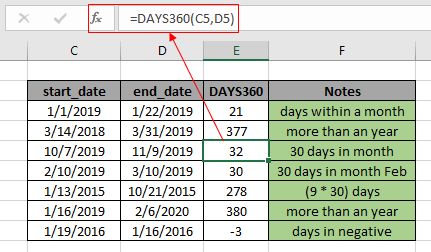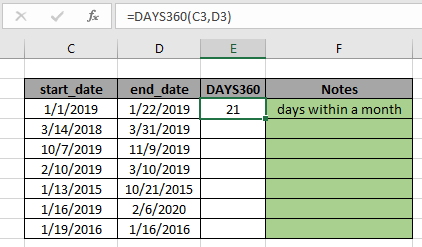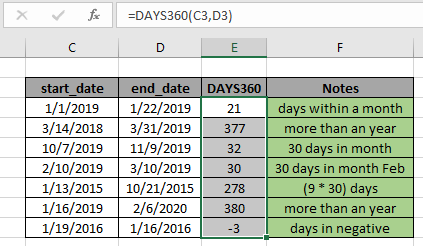# How to use the DAYS360 function in ExcelThe Excel DAYS360 function is a DATE & TIME function. This function takes start date and end date values as input and returns the number of  DAYS between the given values considering 30 days in month in numerical format. In this article, we will learn how to use the DAYS360 function in Excel. DATE value in excel is stored as number. The function considers 30 days in each month for calculation.

Syntax of DAYS360 Function:

=DAYS360(start_date,end_date,[method])

start_date : starting date

end_date : ending date

[method] : [optional] FALSE for U.S.(NASD) method or TRUE for European method

Example of DAYS360 Function:
All of these might be confusing to understand. So, let's test this formula via running it on the example shown below. So, here I have a table of some data. Excel understands time value as numerical value. The first column contains the start_date value, second column contains end_date value and third column has result of the using the DAYS360 function and third column contains the important notes using the function.

Use the formula:

=DAYS360(C3,D3)It will return 21. Here the argument to the DAYS360 function is given using cell reference. The returned value is 21 as excel calculates days between date values starting from the 1/1/2019 to ending upto 1/22/2019. As shown in the above snapshot, you can change the format of any cell to any format using the format cell option. Now You can copy the formula from one to the remaining cells using the Ctrl + D shortcut key or using the drag down option in excel.As you can see from the above snapshot that DAYS360 function takes date value as input and returns the DAYS in numeric value from the given two date values. The function returns #VALUE! Error if the time or date value is invalid.

Here are some observational notes shown below.

Notes:

1. The formula only works with numbers.
2. The function considers 30 days in each month even in february.
3. If the end_date is before the start_date then the result comes with a negative sign. This is the most important feature of the DAYS360 function excel.
4. Arguments to the function must be numeric values or else the function returns #NAME? Error.
5. No argument to the function returns #NUM! Error.
6. The function ignores DAYS360 and DAYS360 value
7. The function returns #VALUE! Error if the date or time value is invalid.

Hope this article about How to use the DAYS360 function in Excel is explanatory. Find more articles on DATE & TIME formulas here. If you liked our blogs, share it with your fristarts on Facebook. And also you can follow us on Twitter and Facebook. We would love to hear from you, do let us know how we can improve, complement or innovate our work and make it better for you. Write to us at info@exceltip.com.

Related Article

minutes to hours and minutes | returns a numerical value which represents the same value but in decimal format in place of time format in excel.

Calculate hours between time in Excel | Calculate the hours between two time values in excel. find the time elapsed between end_time & start_time

Time difference in hours as decimal value | Time difference in hours using Excel MOD function and then we need to represent it in the decimal format.

SUM time over 9 hours in Excel | get the sum of time values which are above 9 hours

How to add Decimal Hours to Time | add numeric or decimal time to a time formatted value.

Popular Articles:

50 Excel Shortcuts to Increase Your Productivity | Get faster at your task. These 50 shortcuts will make you work even faster on Excel.

The VLOOKUP Function in Excel | This is one of the most used and popular functions of excel that is used to lookup value from different ranges and sheets.

COUNTIF in Excel 2016 | Count values with conditions using this amazing function. You don't need to filter your data to count specific values. Countif function is essential to prepare your dashboard.

How to Use SUMIF Function in Excel | This is another dashboard essential function. This helps you sum up values on specific conditions.

Terms and Conditions of use

The applications/code on this site are distributed as is and without warranties or liability. In no event shall the owner of the copyrights, or the authors of the applications/code be liable for any loss of profit, any problems or any damage resulting from the use or evaluation of the applications/code.